Courses
Courses for Kids
Free study material
Free LIVE classes
More

# NCERT Solutions for Class 6 Maths Chapter 14 - Practical GeometryLIVE
Join Vedantu’s FREE Mastercalss

## NCERT Solutions for Class 6 Maths Chapter 14 - Practical Geometry

NCERT solutions for class 6 maths chapter 14 is available in PDF format on the Vedantu. You can download the PDF file for free. The NCERT Solutions Class 6 Maths chapter 14 has been prepared to help students with easy-to-understand explanations. Our subject experts have years of experience in teaching, and their experience is reflected in the solutions they prepared for class 6th practical geometry. If you have any doubts related to class 6 maths chapter 14, you can reach out to our experts. NCERT solutions for class 6 maths chapter 14 practical geometry will help in your exam preparation. Subjects like Science, Maths, English,Hindi will become easy to study if you have access to NCERT Solution for Class 6 Science, Maths solutions and solutions of other subjects.

### Points to Remember before Solving Exercise Problems:

1. Tools used for construction

• Ruler: It is used to draw line segments and measure their lengths. It is a scale on which the length measurements are marked.

• Compass: It is an instrument used to mark lengths and to draw circles and arcs. It has a metal pointer on one end and a pencil holder on the other end.

• Divider: A divider is an instrument having a pair of metal pointers on both ends which are used to compare lengths.

• Set-Squares: Set-Squares are two triangular shaped geometry tools, of which one has 45°, 45°, 90°, and the other has 30°, 60°, 90° angles at the vertices. These are used to draw parallel and perpendicular edges.

• Protractor: It is in a semicircular (D-shape) on which 0o to 180° angles are marked. A protractor is used for drawing and measuring angles.

1. Circle: Circle is a round shape in which all the points on the boundary are at an equal distance from its centre point.

2. A Line Segment: A line segment has a fixed length that can be measured using a ruler. It is a sub-part of a line that has two endpoints.

3. Constructing a Copy of a Line Segment: To do this, we need to use a ruler or both compass and ruler.

4. Perpendicular Lines: If two lines intersect each other in such a way that they make a right angle (90o) at the point of their intersection, then those lines are said to be perpendicular to each other.

5. Constructing Angles: To construct an angle of a given measure we may use a ruler and a protractor.

6. Constructing Angles of Special Measures: There are some other accurate methods to construct some specific angles without using the protractor instead with the help of a compass.

Last updated date: 27th Sep 2023
Total views: 454.8k
Views today: 9.54k

## Access NCERT Solutions for Class 6 Maths Chapter 14 – Practical Geometry

Exercise 14.1

1. Draw a circle of radius $\mathbf{3}.\mathbf{2}$ cm.

Ans. To draw the circle, follow the steps given below:

(i) First, a length of $3.2$ cm is to be measured with the compass.

(Image Will Be Updated Soon)

(ii) Draw a point $'O'$ as the center of the circle at the starting of the considered length.

(Image Will Be Updated Soon)

(iii) Put the pointer of compasses on the point $O$.

(iv) Then, draw the circle by turning the compass slowly around the point $O$, and consider $P$ as the point where the line touches the circle.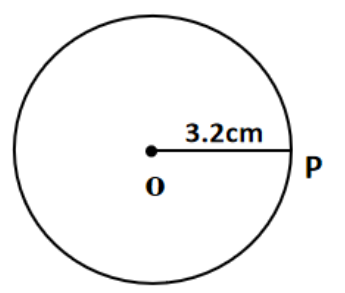2. With the same centre $\mathbf{O}$, draw two circles of radii $\mathbf{4}$ cm and $\mathbf{2}.\mathbf{5}$ cm.

Ans. Follow the procedure given below to make the construction.

(i) Draw a point $'O'$ where we want the circle's centre to be.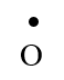(ii) Using the compass, measure a length of $4$ cm.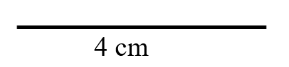(iii) Then, set the compasses' pointer on the point $O$.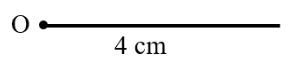(iv) Slowly rotate the compass to draw a circle and let $P$ be the point where the line meets the circle.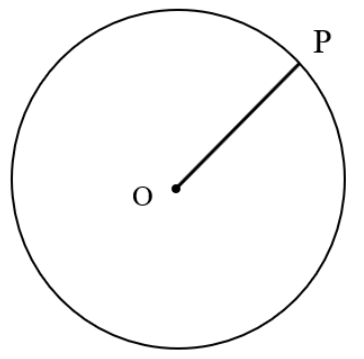(v) Next, using the compass, measure a length of $2.5$ cm.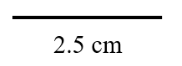(vi) Finally, taking $O$ as the centre, turn the compass slowly around to draw the second circle.

(Image Will Be Updated Soon)

3. Draw a circle and any two of its diameters. If you join the ends of these diameters, what is the figure obtained? What figure is obtained if the diameters are perpendicular to each other? How do you check your answer?

Ans. (i) We get a rectangle by connecting the ends of two diameters of the circle.

$\angle A=\angle B=\angle C=\angle D={{90}^{\circ }}$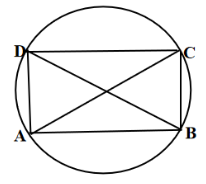(ii) If the diameters of the circle are perpendicular to one other, we can make a square by connecting the ends of the two diameters.

We discover that $AB=BC=CD=DA$ by measuring each side, that is, each of the four sides is equal.

Again, $\angle A=\angle B=\angle C=\angle D={{90}^{\circ }}$, that is, each angle is $90$ degrees.

Thus, it is a square as described in the following figure.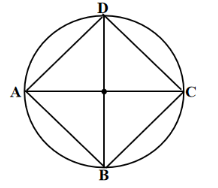4. Draw any circle and mark points $\mathbf{A},\,\mathbf{B},$ and $\mathbf{C}$ such that:

(a) $\mathbf{A}$ is on the circle.

(b) $\mathbf{B}$ is in the interior of the circle.

(c) $\mathbf{C}$ is in the exterior of the circle.

Ans. At first, draw a point $'O'$ where we want the circle's centre to be.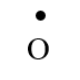Then, sketch a circle of any radius.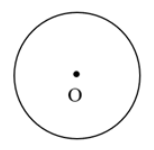Now, draw the points given below.

(a) Point $A$ is on the circle.

(b) Point $B$ is in the interior of the circle.

(c) Point $C$ is on the exterior of the circle.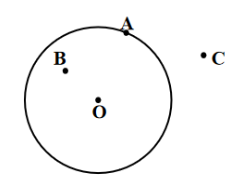5. Let $\mathbf{A}$, $\mathbf{B}$ be the centres of two circles of equal radii; draw them so that each one of them passes through the centre of the other. Let them intersect at $\mathbf{C}$ and $\mathbf{D}$. Examine whether $\overline{\mathbf{AB}}$ and $\overline{\mathbf{CD}}$ are at right angles.

Ans. Draw two equal-radius circles with $A$ and $B$ as their centres, so that one crosses through the centre of the other. The circles intersect at $C$ and $D$. Finally, connect $AB$ and $CD$.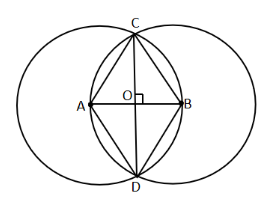Yes, the straight lines $\overline{AB}$ and $\overline{CD}$ intersect each other at right angles.

Exercise 14.2

1.  Draw a line segment of length $\mathbf{7}.\mathbf{3}$ cm, using a ruler.

Ans. Follow the steps given below to complete the construction.

(i) Set the scale's starting point at point $A$.

(ii) At a distance of $7.3$ cm from $A$, draw a point $B$.

(iii) Finally, connect $AB$ using a scale.

Therefore, $AB$ represents the line segment of length $7.3$ cm.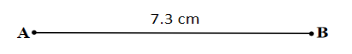2.  Construct a line segment of length $\mathbf{5}.\mathbf{6}$ cm using a ruler and compasses.

Ans. Follow the steps given below to complete the construction.

(i) Draw a line $'l'$. Then, draw a point $A$ on the line.

(ii) Set the scale's starting point at point $A$.

(iii)Then, using the compass, measure 5.6 cm and mark a point B on the line.

Thus, $\overline{AB}$ represents the line segment of length $5.6$ cm as shown in the figure given below.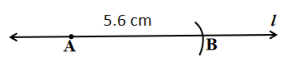3. Construct $\overline{\mathbf{AB}}$ of length $\mathbf{7}.\mathbf{8}$ cm. From this cut off $\overline{\mathbf{AC}}$ of length $\mathbf{4}.\mathbf{7}$ cm. Measure $\overline{\mathbf{BC}}$.

Ans. Follow the steps given below to complete the construction.

(i) First, set the scale's starting point at point $A$.

(ii) Then, at a distance of $7.8$ cm from $A$, draw a point $B$.

(iii) After that, at a distance of $4.7$ cm from $A$, draw a point $C$.

(iv) Finally, measure the length of $BC$ with a scale. The length of $BC=3.1$

cm.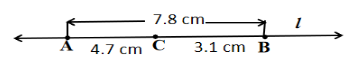4.  Given $\overline{\mathbf{AB}}$ of length $\mathbf{3}.\mathbf{9}$ cm, construct $\mathbf{PQ}$ such that the length $\mathbf{PQ}$ is twice that of $\mathbf{AB}$ .Verify by measurement.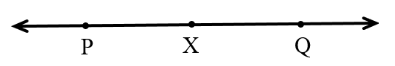(Hint: Construct $\overline{\mathbf{PX}}$ such that length of $\overline{\mathbf{PX}}=$ length of $\mathbf{AB}$; then cut off  $\overline{\mathbf{XQ}}$ such that $\overline{\mathbf{XQ}}$ also has the length of $\overline{\mathbf{AB}}$)

Ans. Follow the steps given below to complete the construction.

(i) First, draw a line $'l'$. Then, $\overline{PX}$ should be constructed so that its length equals the length of $\overline{AB}$, which is $3.9$ cm.

(ii) After that, cut off  $\overline{XQ}$ such that $\overline{XQ}$ also has the length of $\overline{AB}$.

(iii) When the lengths of $\overline{PX}$ and $\overline{XQ}$ are combined together, they equal double the length of $\overline{AB}$.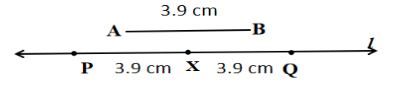Verification:

By measuring the lengths, it is found that, $\overline{PQ}=7.8$ cm

$=3.9$ cm $+$$3.9$ cm

$=\overline{AB}+\overline{AB}$

$=2\times AB$.

5. Given $\overline{\mathbf{AB}}$ of length $\mathbf{7}.\mathbf{3}$ cm and $\overline{\mathbf{CD}}$ of length $\mathbf{3}.\mathbf{4}$ cm, construct a line segment $\overline{\mathbf{XY}}$ such that the length of $\overline{\mathbf{XY}}$ is equal to the difference between the lengths of $\overline{\mathbf{AB}}$ and $\overline{\mathbf{CD}}$. Verify by measurement.

Ans. Follow the steps given below to complete the construction.

(i) First, draw a line $'l'$. Then, mark a point $X$ on the line.

(ii) Make the line $\overline{XZ}$ so that the length of $\overline{XZ}$ equals the length of $\overline{AB}$, which is $7.3$ cm.

(iii) After that, cut $\overline{ZY}=$ length of $\overline{CD}=3.4$.

(iv) Hence, the length of line $\overline{XY}=$ length of $\overline{AB}-$ length of $\overline{CD}$.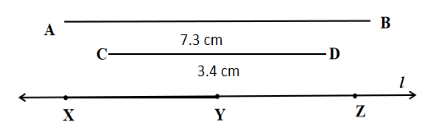Verification:

By measuring the lengths, it is found that, length of $XY=3.9$ cm

$=7.3$ cm $-$ $3.4$ cm

$=\overline{AB}-\overline{CD}$.

Exercise 14.3

1. Draw any line segment $\overline{\mathbf{PQ}}$. Without measuring $\overline{\mathbf{PQ}}$, construct a copy of $\overline{\mathbf{PQ}}$.

Ans. Follow the steps given below to complete the construction.

(i) First, set the compass pointer on $P$ and take a measurement of point $Q$. The length of $\overline{PQ}$ is now determined by the instrument's opening.

(ii) Then, draw a line $'l'$. Mark a point $A$ on the line $'l'$. Place the pointer on the point $A$ without modifying the compass settings.

(iii) Make an arc which cuts $'l'$ at a point $B$. Thus, $\overline{AB}$ becomes a copy of the line $\overline{PQ}$.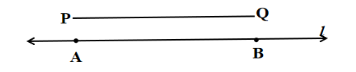2. Given some line segment $\overline{\mathbf{AB}}$, whose length you do not know, construct $\overline{\mathbf{PQ}}$ such that the length of $\overline{\mathbf{PQ}}$ is twice that of $\overline{\mathbf{AB}}$.

Ans. Follow the steps given below to complete the construction.

(i) Length of $\overline{AB}$ is not known.

(ii) Place the compass pointer on $A$ and take a measurement of $B$. The length of $\overline{AB}$ is now determined by the instrument's opening.

(iii) Draw a line $'l'$. Mark a point $P$ on $'l'$. Place the pointer on $Q$ without changing the compass setting.

(iv) Make an arc which cuts $'l'$ at a point $R$.

(v) Now, by placing the pointer on $R$, draw another arc that cuts $'l'$ at point $Q$ without changing the compass setting..

$\overline{PQ}$ is the needed line segment, which is twice as long as $\overline{AB}$.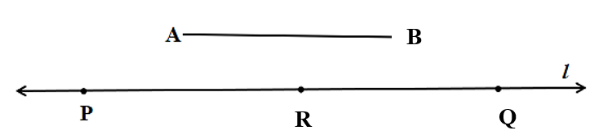Exercise 14.4

1. Draw any line segment $\overline{\mathbf{AB}}$ . Mark any point $\mathbf{M}$ on it. Through $\mathbf{M}$, draw a perpendicular to $\overline{\mathbf{AB}}$. (Use ruler and compasses).

Ans. Follow the steps given below to complete the construction.

(i) Construct an arc intersecting the line $\overline{AB}$ at two points $C$ and $D$, taking $M$ as the centre.

(ii) Draw two arcs with $C$ and $D$ as centres and a radius bigger than

$MC$. Point your compass on $C$ and construct an arc, then point your compass on $D$ and draw another arc, cutting each other at $P$.

(iii) Finally, connect the line $PM$. Then, through the point $M$, $PM$ is perpendicular to $AB$ as the figure below.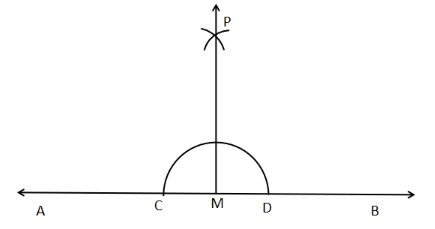2. Draw any line segment $\overline{\mathbf{PQ}}$. Take any point $\mathbf{R}$ not on it. Through $\mathbf{R}$, draw a perpendicular to $\overline{\mathbf{PQ}}$. (Use ruler and set-square).

Ans. Follow the steps given below to complete the construction.

(i) Place a set-square on $\overline{PQ}$ so that one of its right-angle sides is aligned with $\overline{PQ}$.

(ii) Place a ruler along the edge of the set-square that is opposite the right angle.

(iii) Join $\overline{RM}$ along the side through $R$, where it will meet $\overline{PQ}$ at $M$. Then $\overline{RM}$ will be perpendicular to $\overline{PQ}$.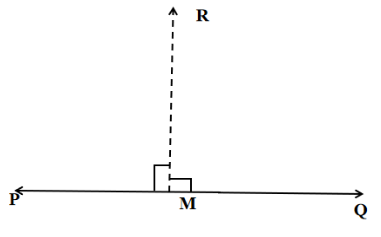3. Draw a line $l$ and a point $\mathbf{X}$ on it. Through $\mathbf{X}$, draw a line segment $\overline{\mathbf{XY}}$ perpendicular to $l$. Now draw a perpendicular to$\overline{\mathbf{XY}}$ to $\mathbf{Y}$. (use ruler and compasses).

Ans. Follow the steps given below to complete the construction.

(i) Draw any line $'l'$ and then mark a point $X$ on it.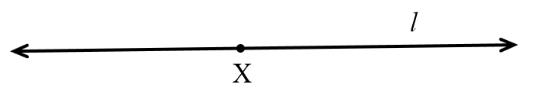(ii) Make an arc taking $X$ as the centre, which intersects the line $'l'$ at two points $A$and $B$.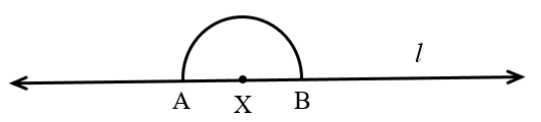(iii) Now, taking $A$ and $B$ as the centres and a radius longer than $\overline{XA}$, sketch two arcs that meet at the point $P$.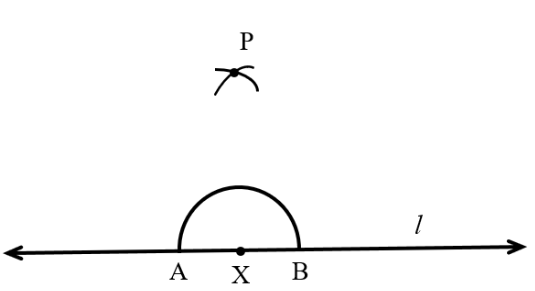(iv) After that, connect the line $\overline{XP}$ and extend it to $Y$. Then $\overline{XY}$ becomes perpendicular to $'l'$.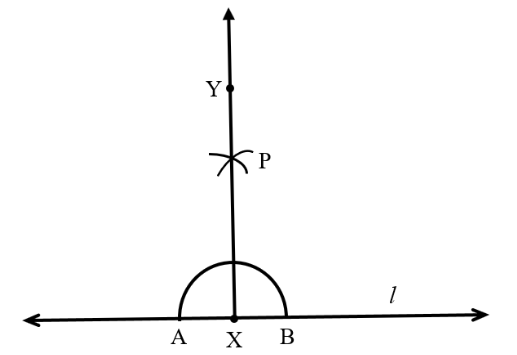(v) Construct an arc with $Y$ as the centre that intersects $\overline{XY}$ at the points $C$ and $D$.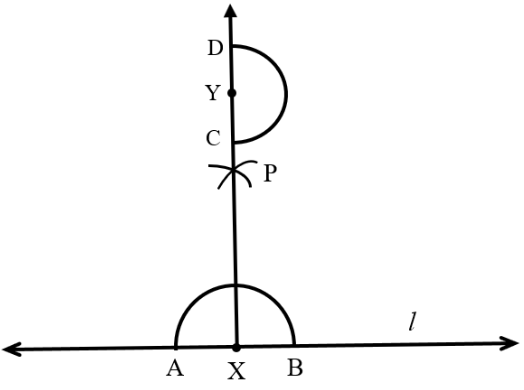(vi) Draw two arcs that intersect at $F$, taking $C$ and $D$ as centres and a radius longer than $\overline{YD}$.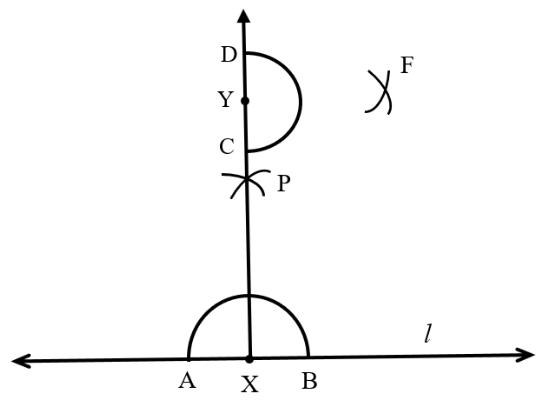(vii) Finally, connect the line $\overline{YF}$, and then $YF$ becomes perpendicular to $\overline{XY}$ at $Y$, as shown in the figure given below.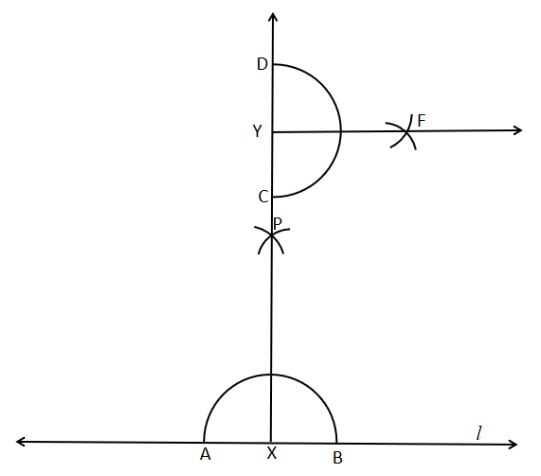Exercise 14.5

1. Draw $\overline{\mathbf{AB}}$ of length $\mathbf{7}.\mathbf{3}$ cm and find its axis of symmetry.

Ans. The axis of symmetry of the line segment $\overline{AB}$ will serve as the perpendicular bisector of $\overline{AB}$. As a result, the perpendicular bisector of $\overline{AB}$ must be drawn.

Now, follow the steps given below to complete the construction.

(i) First, draw any line segment $\overline{AB}$ of length $7.3$ cm.

(ii) Then, construct two arcs that meet at $C$ and $D$, considering $A$ and $B$ as centres and a radius that is longer than half of $\overline{AB}$.

(iii) After that, connect the line $\overline{CD}$.

Thus, $\overline{CD}$ becomes the axis of symmetry of the line segment $\overline{AB}$ such that $\overline{AO}=\overline{BO}$ as shown in the figure given below.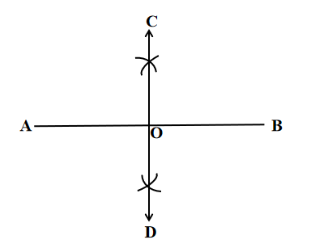2. Draw a line segment of length $\mathbf{9}.\mathbf{5}$ cm and construct its

perpendicular bisector.

Ans. Follow the steps given below to complete the construction.

(i) First, draw any line segment $\overline{AB}$ of length $9.5$ cm

(ii) Then, construct two arcs that meet at $C$ and $D$, considering $A$ and $B$ as centres and a radius that is longer than half of $\overline{AB}$.

(iii) After that, connect $\overline{CD}$ using the scale.

Thus, $\overline{CD}$ becomes the perpendicular bisector of $\overline{AB}$ such that $\overline{AO}=\overline{BO}$ as shown in the figure given below.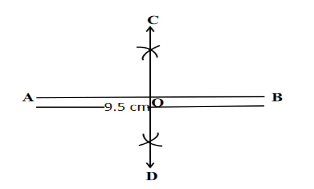3. Draw the perpendicular bisector of $\overline{\mathbf{XY}}$ whose length is $\mathbf{10}.\mathbf{3}$ cm.

(a) Take any point $\mathbf{P}$ on the bisector drawn. Examine whether $\overline{\mathbf{PX}}=\overline{\mathbf{PY}}$.

Ans. Follow the steps given below to complete the construction.

(i) First, draw any line segment $\overline{XY}$ of length $10.3$ cm.

(ii) Then, construct two arcs that meet at $C$ and $D$, considering $X$ and $Y$ as centres and a radius that is longer than half of $\overline{AB}$.

(iii)After that, connect $\overline{CD}$ by using a scale such that it intersects $\overline{XY}$ at $P$.

Thus, $\overline{CD}$ becomes the perpendicular bisector of the line segment $\overline{XY}$ so that, $\overline{PX}=\overline{PY}$ as shown in the figure given below.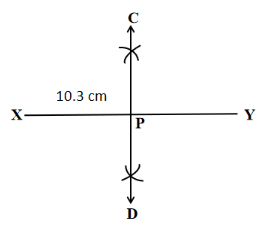(b) If $\mathbf{M}$ is the mid-point of $\mathbf{XY}$ , what can you say about the lengths $\mathbf{MX}$ and $\mathbf{XY}$?

Ans. Follow the steps given below to complete the construction.

(i) First, draw any line segment $\overline{XY}$  of length $10.3$ cm.

(ii) Then, construct two arcs that meet at $C$ and $D$, considering $X$ and $Y$ as centres and a radius that is longer than half of $\overline{AB}$.

(iii) After that, connect $\overline{CD}$ by using a scale which intersects $\overline{XY}$ at $M$.

(iv) Thus, $\overline{CD}$ becomes the perpendicular bisector of the line segment $\overline{XY}$ so that, $\overline{MX}=\overline{MY}$.

(v) Hence, $M$ is the midpoint of the line segment $\overline{XY}$ and so, $\overline{MX}=\frac{1}{2}\overline{XY}$ as shown in the figure given below.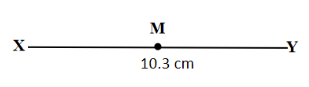4. Draw a line segment of length $\mathbf{12}.\mathbf{8}$ cm. Using compasses, divide it into four equal parts. Verify by actual measurement.

Ans. Follow the steps given below to complete the construction.

(i) First, draw any line segment $\overline{AB}$ of length $12.8$ cm.

(ii) Then, construct the perpendicular bisector of $\overline{AB}$, which intersects it at the point $C$.

(iii) Therefore, C becomes the mid-point of the line-segment $\overline{AB}$.

(iv) After that, construct the perpendicular bisector of $\overline{AC}$ that intersects it at the point $D$. So, $D$ becomes the mid-point of the line-segment $\overline{AC}$.

(v) Again, construct the perpendicular bisector of the segment $\overline{CB}$ that intersects it at the point $E$.

(vi) Then, $E$ becomes the mid-point of the line-segment $\overline{CB}$.

Thus, the points $C,\,\,D,$ and $E$ divide $\overline{AB}$ in four equal parts.

Hence, by measuring the lengths, it is found that, $\overline{AD}=\overline{DC}=\overline{CE}=\overline{EB}=3.2$ cm.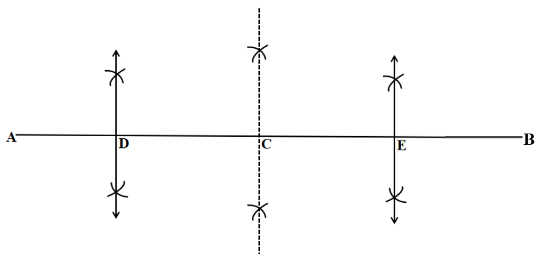5. With $\overline{\mathbf{PQ}}$ of length $\mathbf{6}.\mathbf{1}$ cm as diameter, draw a circle

Ans. Follow the steps given below to complete the construction.

(i) First, draw any line segment $\overline{PC}$ of length $6.1$ cm.

(ii) Then, construct a perpendicular bisector of the segment $\overline{PQ}$ that intersects it at the point $O$.

(iii)Therefore, $O$ becomes the mid-point of the line-segment $\overline{PQ}$.

(iv)Finally, construct a circle with $O$ as the centre and $\overline{OP}$ or $\overline{OQ}$ as the radius, with the diameter being the line segment $\overline{PQ}$.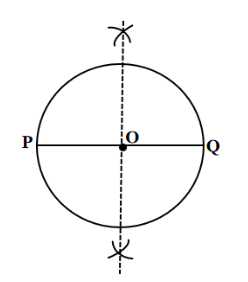6. Draw a circle with centre $\mathbf{C}$ and radius $\mathbf{3}.\mathbf{4}$ cm. Draw any chord $\overline{\mathbf{AB}}$. Construct the perpendicular bisector $\overline{\mathbf{AB}}$ and examine if it passes through $\mathbf{C}$.

Ans. Follow the steps given below to complete the construction.

(i) First, construct a circle taking $C$ as centre and the radius of length $3.4$ cm.

(ii) Then, draw any chord $\overline{AB}$.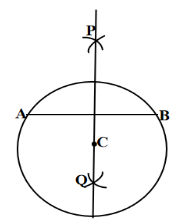(iii) After that, construct two arcs that meet at $P$ and $Q$, considering $A$ and $B$ as centres and a radius that is longer than half of $\overline{AB}$.

(iv) Finally, connect $\overline{PQ}$.

Hence, $\overline{PQ}$ becomes the perpendicular bisector of the line segment $\overline{AB}$ such that it passes through the point $C$.

7. Repeat Question $\mathbf{6}$, if $\mathbf{AB}$ happens to be a diameter.

Ans. Follow the steps given below to complete the construction.

(i) First, construct a circle taking $C$ as centre and the radius of length $3.4$ cm.

(ii) Then, draw a diameter $\overline{AB}$.

(iii) After that construct two arcs that meet at $P$ and $Q$, considering $A$ and $B$ as centres and a radius that is longer than half of $\overline{AB}$.

Next connect $\overline{PQ}$.

Thus, $\overline{PQ}$ becomes the perpendicular bisector of the segment $\overline{AB}$.

It can be noticed that the perpendicular bisector $\overline{PQ}$ of $\overline{AB}$ passes through the centre $C$ of the circle.8. Draw a circle of radius $\mathbf{4}$ cm. Draw any two of its chords. Construct the perpendicular bisectors of these chords. Where do they meet?

Ans. Follow the steps given below to complete the construction.

(i) First, construct a circle taking $O$ as the centre and the radius of length $4$ cm.

(ii) Then, draw any two chords $\overline{AB}$ and $\overline{CD}$ in the circle.

(iii) After that, construct two arcs that meet at $E$ and $F$, considering $A$ and $B$ as centres and a radius that is longer than half of $\overline{AB}$.

(iv) Next, connect $\overline{EF}$ by using a scale.

Thus, $\overline{EF}$ becomes the perpendicular bisector of the chord $\overline{AB}$.

(v) Similarly, construct the perpendicular bisector $\overline{GH}$ of the chord $\overline{CD}$.

Hence, $\overline{EF}$ and $\overline{GH}$, two perpendicular bisectors, intersect at the centre $O$ as shown in the figure given below.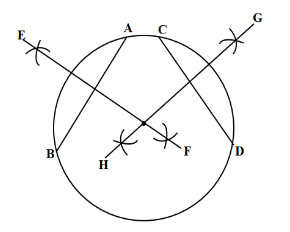9. Draw any angle with the vertex $\mathbf{O}$. Take a point $\mathbf{A}$ on one of its arms and $\mathbf{B}$ on another such that $\mathbf{OA}=\mathbf{OB}$. Draw the perpendicular bisectors of $\overline{\mathbf{OA}}$ and $\overline{\mathbf{OB}}$. Let them meet at $\mathbf{P}$. Is$\mathbf{PA}=\mathbf{PB}$?

Ans. Follow the steps given below to complete the construction.

(i) First, draw an angle taking $O$ as the vertex.

(ii) Then, consider a point $A$ on one arm and $B$ on another, so that $OA=OB$.

(iii) After that, construct perpendicular bisectors on the segments $\overline{OA}$ and $\overline{OB}$ such that they intersect at $P$.

(iv) Next, connect $\overline{PA}$ and $\overline{PB}$.

(v) Finally, measure the lengths with a scale and verify whether $PA=PB$.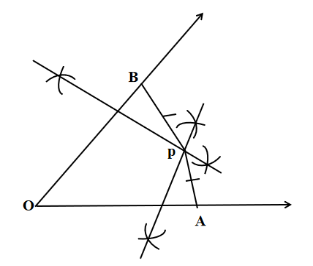Exercise 14.6

1. Draw $\angle \mathbf{POQ}$ of measure $\mathbf{7}{{\mathbf{5}}^{\mathbf{o}}}$ and find its line of symmetry.

Ans. Follow the steps given below to complete the construction.

(i) First, draw any line $l$ and take a point of $O$ on $l$.

(ii) Then, set the compass's pointer at the point $O$ and then draw an arc that meets the line $l$ at a point $A$.

(iii)After that, cut the previous arc at $B$, keeping the same radius and centre $A$.

(iv)Next, connect $OB$. Therefore, the angle becomes, $\angle BOA={{60}^{\circ }}$.

(v) Then, cut the previous arc at $C$, keeping the same radius and centre $B$.

(vi) After that, construct a bisector of the angle $\angle BOC$. Let the bisector intersect the arc at $D$. So, the angle $\angle DOA={{90}^{o}}$.

(vii)Finally, construct a bisector $OP$ of the angle $\angle DOB$. Hence, the required angle obtained is $\angle POA={{75}^{\circ }}$.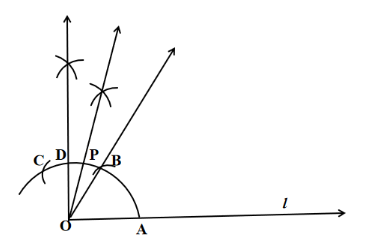2. Draw an angle of measure $\mathbf{14}{{\mathbf{7}}^{\mathbf{o}}}$ and construct its bisector.

Ans. Follow the steps given below to complete the construction.

(i) First, draw any line $OA$.

(ii) Then, draw the angle $\angle AOB={{147}^{\circ }}$ by using a protractor.

(iii) After that, considering $O$ as the centre, construct an arc that meets the arms $\overline{OA}$  and $\overline{OB}$ at the points $P$ and $Q$ respectively.

(iv) Now, construct an arc considering $P$ as centre and with a radius that is longer than half of $PQ$.

(v) Also, construct another arc considering $Q$ as centre and a radius that is longer than half of $PQ$ such that both the arcs meet at $R$.

(vi) Next, connect $\overline{OR}$.

Thus, $\overline{OR}$ becomes the needed bisector of the angle $\angle AOB$.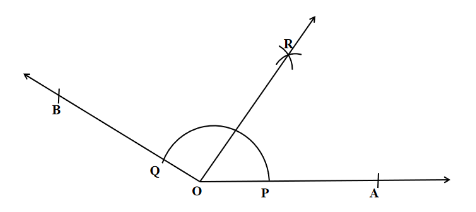3. Draw a right angle and construct its bisector

Ans. Follow the steps given below to complete the construction.

(i) First, draw any line $PQ$ and mark a point $O$ on $PQ$.

(ii) Then, construct an arc that meets $PQ$ at the points $A$ and $B$, considering $O$ as the centre and a convenient radius.

(iii) Draw two arcs that meet at $C$, considering $A$ and $B$ as centres and a radius greater than half of $AB$.

(iv) After that, connect $OC$. Therefore, the angle $\angle COQ$ becomes the needed right angle.

(v) Now, draw two arcs that meet at $D$, considering $E$ and $B$ as centres and a radius greater than half of $BE$.

(vi)Then, connect $OD$.

Hence, $OD$ becomes the needed bisector of the right-angle $\angle COQ$.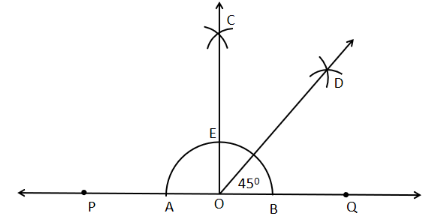4. Draw an angle of measure $\angle \,\mathbf{15}{{\mathbf{3}}^{\mathbf{o}}}$ and divide it into four equal parts.

Ans. Follow the steps given below to complete the construction.

(i) First, draw a straight line $OA$.

(ii) Then, draw an angle $\angle AOB={{153}^{\circ }}$ at the point $O$, by using a protractor.

(iii) After that, construct the bisector $OD$ of the angle $\angle AOB$.

(iv) Again, construct the bisector $OC$ of the angle $\angle AOD$.

(v) Also, construct the bisector $OE$ of the angle $\angle BOD$.

Thus, the angle $\angle AOB={{153}^{\circ }}$ is divided in four equal parts by the bisectors $OC$, $OD$ and $OE$ as shown in the figure given below.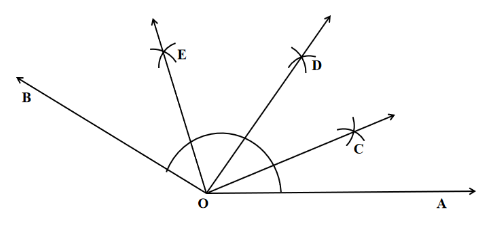5. Construct the following angles with a ruler and compasses.

(a) $\mathbf{6}{{\mathbf{0}}^{\mathbf{o}}}$

Ans. Follow the steps given below to complete the construction.

First, draw a straight line $OA$.

(i) Then, considering the centre as $O$, construct an arc that meets $OA$ at the point $P$.

(ii) After that, consider the centre as $P$ and with the same radius, construct another arc which meets the previous arc at the point $Q$.

(iii) Then, connect $OQ$.

Hence, the needed angle is $\angle BOA={{60}^{\circ }}$.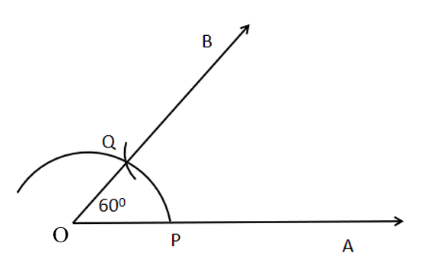(b) $\mathbf{3}{{\mathbf{0}}^{\mathbf{o}}}$

Ans. Follow the steps given below to complete the construction.

(i) First, draw any straight line $OA$.

(ii) Then, considering $O$ as the centre, draw an arc that meets $OA$ at the point $P$.

(iii) Similarly, considering  $P$ as the centre and with the same radius, draw another arc that intersects the previous arc at the point $Q$.

(iv) After that, connect $OQ$.

(v) Thus, $\angle BOA$ becomes the angle ${{60}^{o}}$.

(vi) Now, set the compass’s pointer on the point $P$ and taking a radius greater than $PQ$, draw an arc.

(vii) Again, set the compass’s pointer on the point $Q$ without changing the radius, draw another arc that intersects the previous arc at the point $C$.

Hence, connecting $OC$, the needed angle obtained is $\angle COA={{30}^{\circ }}$.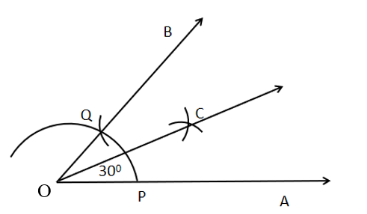(c) $\mathbf{9}{{\mathbf{0}}^{\mathbf{o}}}$

Ans. Follow the steps given below to complete the construction

(i) First, draw any line $OA$.

(ii) Then, considering $O$ as the centre, draw an arc that meets $OA$ at the point $X$.

(iii) Similarly, considering  $X$ as the centre and with the same radius, draw another arc that intersects the previous arc at the point $Y$.

(iv)Again, considering  $Y$ as the centre and with the same radius, draw another arc that intersects the previous arc at the point $Z$.

(v) Now, considering $Y$ and $Z$ as centers and with the same radius, draw two arcs that will meet at the point $S$.

(vi) Then, connect the OS and extend it to construct $OB$.

Hence, the needed angle is $\angle BOA={{90}^{\circ }}$.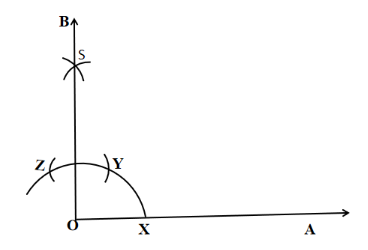(d) $\mathbf{12}{{\mathbf{0}}^{\mathbf{o}}}$

Ans. Follow the steps given below to complete the construction.

(i) First, draw any line $OA$.

(ii) Then, considering the point $O$ as the centre, draw an arc that meets $OA$ at the point $P$.

(iii) Again, considering the point $P$ as the centre and with not changing the radius, draw an arc that meets the previous arc at the point $Q$.

(iv) Also, considering the point $Q$ as the centre and with the same radius, draw an arc that meets the same previous arc at the point $S$.

Now, connect $OS$.

(v) Hence, the needed angle is $\angle AOD={{120}^{\circ }}$.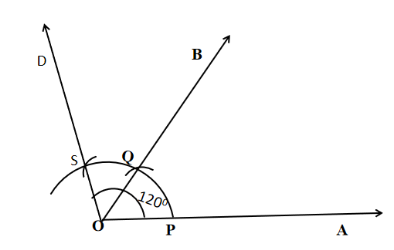(e) $\mathbf{4}{{\mathbf{5}}^{\mathbf{o}}}$

Ans. Follow the steps given below to complete the construction.

(i) First, draw any straight line $OA$.

(ii) Then, considering the point $O$ as the centre, draw an arc that meets $OA$ at the point $X$.

(iii) Similarly, considering the point $X$ as the centre and with not changing the radius, draw an arc that meets the previous arc at the point $Y$.

(iv) Again, considering the point $Y$ as the centre and with the same radius, draw an arc that meets the same previous arc at the point $Z$.

(v) Also, considering $Y$ and $Z$ as centers and with the same radius, draw two arcs that will meet at the point $S$.

(vi) Then, connect $OS$ and extend it to construct $OB$.

Thus, $\angle BOA$ becomes that angle ${{90}^{\circ }}$.

(vii) Now, construct the bisector $OM$ of the angle $\angle BOA$.

Hence, the needed angle is $\angle MOA={{45}^{\circ }}$.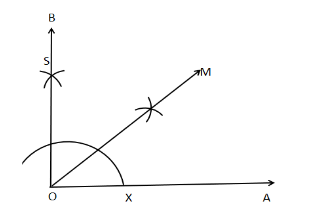(f) $\mathbf{13}{{\mathbf{5}}^{\mathbf{o}}}$

Ans. Follow the steps given below to complete the construction.

(i) First, draw any straight line $PQ$ and mark a point $O$ on $PQ$.

(ii) Then, considering the point $O$ as the centre, draw an arc that meets $PQ$ at the points $A$ and $B$.

(iii) Then, considering the points $A$ and $B$ as centers and with the radius greater than half of $AB$, draw two arcs that will meet at the point $R$.

(iv) Now, connect $OR$. So, we have, $\angle QOR=\angle POQ={{90}^{\circ }}$.

(v)Then construct the bisector $OD$ of the angle $\angle POR$.

Hence, the needed angle is $\angle QOD={{135}^{\circ }}$.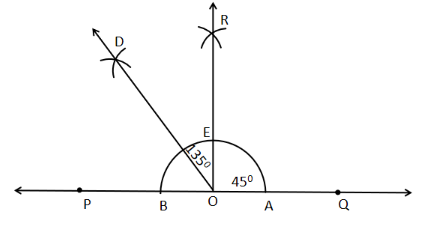6. Draw an angle of measure $\mathbf{4}{{\mathbf{5}}^{\mathbf{o}}}$ and bisect it.

Ans. Follow the steps given below to complete the construction.

(i) First, draw any straight line $PQ$ and mark any point $O$ on $PQ$.

(ii) Then, considering the point $O$ as the centre, draw an arc that meets $PQ$ at the points $A$ and $B$.

(iii) After that, considering the points $A$ and $B$ as centers and with the radius greater than half of $AB$, draw two arcs that will meet at the point $C$.

(iv) Now, connect to OC. Therefore, the angle $\angle COQ$ becomes ${{90}^{\circ }}$.

(v) Then, construct the bisector $OE$ of angle $\angle COQ$. So,$\angle QOE={{45}^{\circ }}$.

(vi)Also, construct the bisector $OG$ of the angle $\angle QOE$.

Hence, the required bisected angle is $\angle QOG=\angle EOG={{22.5}^{\circ }}$.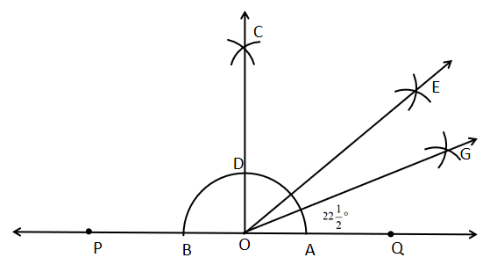7. Draw an angle of measure $\mathbf{13}{{\mathbf{5}}^{\mathbf{o}}}$ and bisect it.

Ans. Follow the steps given below to complete the construction.

(i) First, draw any straight line $PQ$ and mark a point $O$ on $PQ$.

(ii) Then, considering the point $O$ as the centre, draw an arc that meets $PQ$ at the points $A$ and $B$.

(iii) After that, considering the points $A$ and $B$ as centers and with the radius greater than half of $AB$, draw two arcs that will meet at the point $R$.

(iv) Now, connect $OR$. Therefore, we have, $\angle QOR=\angle POQ={{90}^{\circ }}$.

(v) Then, construct the bisector $OD$ of the angle $\angle POR$.

Thus, the angle $\angle QOD={{135}^{\circ }}$.

(vi)Again, construct the bisector $OE$ of the angle $\angle QOD$.

Hence, the required bisected angle is $\angle QOE=\angle DOE={{67.5}^{\circ }}$.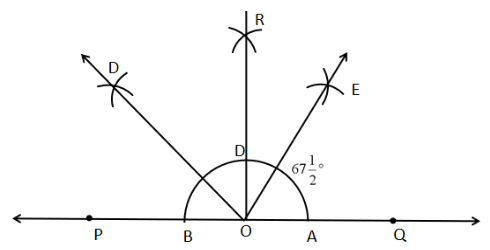8. Draw an angle of $\mathbf{7}{{\mathbf{0}}^{\mathbf{o}}}$. Make a copy of it using only a straight edge and compasses.

Ans. Follow the steps given below to complete the construction.

(i) First, construct an angle $\angle POQ={{70}^{\circ }}$.

(ii) Then, draw any straight line $AB$.

(iii) After that, set the compass’s pointer at the point $O$ and mark an arc to intersect the lines of the angle $\angle POQ$ at the points $L$ and $M$.

(iv) Next, mark another arc taking $A$ as centre by not changing the last used setting of the compass such that the arc intersects $AB$ at the point $X$.

(v) Now, make the radius of the compass setting the same as the length of $LM$.

(vi) Then, set the pointer of the compass at the point $X$ and mark an arc that will intersect the previously drawn arc at the point $Y$.

(vii) Therefore, connecting $AY$ by using a scale, the required angle is obtained as $\angle YAX={{70}^{\circ }}$.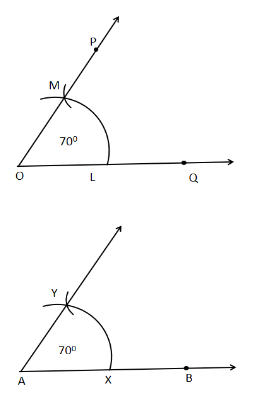9. Draw an angle of $\mathbf{4}{{\mathbf{0}}^{\mathbf{o}}}$. Copy its supplementary angle.

Ans. Follow the steps given below to complete the construction.

(i) First, construct an angle $\angle AOB={{40}^{\circ }}$.

(ii) Then, draw any straight line $PQ$ and mark a point $M$ on it.

(iii) After that, set the compass’s pointer at the point $O$ and mark an arc to intersect the lines of the angle $\angle AOB$ at the points $L$ and $N$.

(iv) Next, mark another arc taking $M$ as centre by not changing the last used setting of the compass such that the arc intersects $MQ$ at the point $X$.

(v) Now, make the radius of the compass setting the same as the length of $LN$.

(vi) Then, set the pointer of the compass at the point $X$ and mark an arc that will intersect the previously drawn arc at the point $Y$.

(vii) Therefore, connect $MY$ by using a scale such that the angle $\angle QMY={{40}^{\circ }}$.

Hence, the supplementary angle $\angle PMY={{180}^{\circ }}-{{40}^{\circ }}={{140}^{\circ }}$.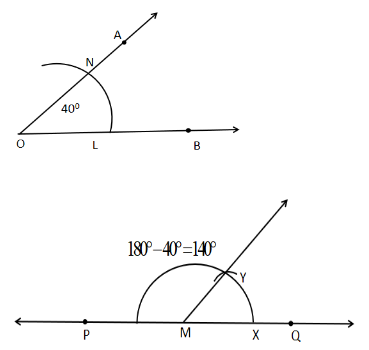Vedantu provides the most reliable Solutions to the NCERT class 6 maths chapter 14. The NCERT chapter 14 practical geometry class 6 solutions are prepared by our subject experts in a step-by-step method. Students can download the NCERT solution for class 6 maths chapter 14 practical geometry PDF at their convenience, for studying offline as well.

## NCERT Solutions for Class 6 Maths Chapters

### Introduction

In the NCERT solutions, the first section deals with the different concepts of how to make different shapes of definite measurements. The NCERT solutions for class 6 maths chapter 14 practical geometry also present a list of different problems and their solutions to learn these concepts. You will also find some unsolved problems at the end of the chapter for you to practice. The problems shown in practical geometry class 6 PDF are based on various difficulty levels that will help you develop a conceptual understanding. Vedantu experts have prepared the class 6 maths practical geometry solutions in an easily understandable manner.

## The Circle

All of you know what a circle is. It would be best to remember that a circle is a body with no corners. The distance of all the points on a circle is the same from its center. The distance between the center of a circle and any point on the circle is called the radius. Once you know the radius of a circle, you can easily draw the circle. You will get the detailed steps of constructing a circle with a known radius in the NCERT solutions for class 6 maths chapter 14 practical geometry. For example, if the radius is 6 cm, you need to put the tip of your compass at the 0th mark and the pencil attached to it at the ruler's 6 cm mark. Then you need to place the tip at the center of the circle and draw the circle. Make sure you do not move the tip of the compass while you are drawing the circle. Otherwise, your circle will have an unequal radius in different regions.

You must have developed some concepts until now. You need to use these concepts to solve the problems given at the end of class 6th maths chapter 14. To find out the solutions for class 6 maths chapter 14, you can download the PDF file and go through the similar solutions depicted in the file.

Exercise 14.1 Solutions: 5 Questions (2 short questions and 3 Long questions).

## A Line Segment

If you have studied lines in previous classes, you must remember the difference between a line and a line segment. A line has no endpoints. A part of a line is called a line segment. The line segment has two endpoints. Therefore, there is a defined length of a line segment. If you know the length of a line segment, you can easily draw a line segment. The process of drawing a line segment of a defined length is discussed in detail in the NCERT solutions for class 6 maths chapter 14. For example, the length of the line segment is 5 cm. For drawing a line segment, you need a pencil and a ruler. You need to mark the starting point of the line segment on the paper. Then you need to use your ruler and place another point 5 cm away from the previous point. These are the two endpoints of your line segment. Now you need to join the two points with a straight line, and you must do it with the help of the ruler again. In this way, you can draw a line segment of a definite length. You will learn about this technique in detail in NCERT solutions for class 6 maths chapter practical geometry.

Constructing a line segment is one of the easiest things to learn in practical geometry. You can easily draw it with a pencil and a ruler. If you want to solve problems on this topic, you must follow ch 14 maths class 6 NCERT solutions provided on Vedantu.

Exercise 14.2 Solutions: 5 Questions (5 short questions).

Copying a line segment is as easy as drawing one. You can follow simple techniques like tracing it with the help of a tracing paper. You can also measure the line segment's length and then draw the next one of the same length. However, if you want to copy the line segment with a ruler and a compass, you should refer to NCERT solutions for class 6 maths chapter 14. Take the compass and put on the two ends of the line segment to be copied. Then put the compass on any point on another line. Use the pencil attached to the compass to make a mark on the line, with the measured length. Erase out the rest of the line. This line segment thus formed is of the same length as that of the previous line segment.

Copying a line segment is easy to do if you follow the proper instructions mentioned in the NCERT Solutions for class 6 maths chapter 14 practical geometry. Follow the details mentioned in the PDF to learn the easy steps.

Exercise 14.3 Solutions: 2 Questions (2 long questions).

## Perpendiculars

Drawing a perpendicular on a definite point on the line is easy. You can do it by choosing the point and then using the set square on that point. The set square will give you a perfect perpendicular. If the point is not on the line, use a compass to draw an arc on the line to cross in two points. Now take the compass on both the points, one at a time, and draw arcs on the other side of the line. Join the point on th straight line and the point of intersection of both the arcs and the line will be perpendicular to the previous line. You can also make the perpendiculars by the paper folding technique shown in  NCERT solutions for class 6th maths chapter 14.

You can learn the construction of perpendicular if you go through practical geometry class 6 NCERT solutions.

Exercise 14.4 Solutions: 3 Questions (3 Long questions).

If you want to draw one perpendicular bisector of a line segment, put your compass at each end of the line segment and draw a semi-circle with a radius greater than half of the line segment, from each end. There will be two intersection points of these two circles. Join them with a straight line, and you will get the perpendicular bisector.

You can easily draw perpendicular bisectors on line segments by following the rules mentioned in class 6 maths practical geometry NCERT Solutions.

Exercise 14.5 Solutions: 9 Questions (9 Long questions).

## Angles

You can learn different techniques of constructing angles in practical geometry class 6 NCERT solutions. You can draw the angle of a given measure by placing a protractor on the line segment and marking the angle measure. Draw a line from the end of the base line-segment to the marked point.

If you want to copy an unknown measure angle, draw a separate line segment and mark a point. Use the compass to make an arc on the given angle to cross both the lines. Without disturbing the compass angle, draw another arc on the new line segment with the new point as the center. Then go back to the previous angle, put the compass point and the pencil on the intersection points of the arc on the angle, respectively. Using the same measurement, draw another arc on the new line to intersect the previous arc. Joining the two arcs' intersection point with the point on the new line will copy the angle.

If you want to bisect an angle, take the compass, draw one arc with the center's meeting point, and the arc meets both the lines. Put the compass on the intersection point on one line of the angle so that the pencil is more than halfway but does not reach the other line. Follow the same with the other intersection point. The meeting point of both the arcs is the angle bisector.

You can also create angles of 60, 30, 90, and 120 degrees, in this way. You do not need the protractor for this purpose. You can make it with the compass and the ruler. You can also create angles half of these values by following the technique to draw an angle bisector. All these techniques are mentioned in ch 14 maths class 6 NCERT solutions.

You can easily draw angles of various kinds by following the procedure mentioned in class 6 maths chapter 14 NCERT solutions.

Exercise 14.6 Solutions: 9 Questions (1 short question and 8 Long questions).

### Key Features of NCERT Solutions for Class 6 Maths Chapter 14

The key features of class 6 practical geometry NCERT solutions will include:

• Easy to understand approaches for the students.

• The topics cover concepts required for all examinations.

• The explanations will provide a basic understanding to solve complex problems.

### Chapter wise NCERT Solutions for Class 6 Maths

Along with this, students can also view additional study materials provided by Vedantu, for Class 6 Maths Chapter 1 Knowing Our Numbers

## FAQs on NCERT Solutions for Class 6 Maths Chapter 14 - Practical Geometry

1. How will I Draw a Circle of a Definite Radius?

You can draw the circle of a definite radius using a compass, a ruler, and a pencil. First, measure the radius on the compass from the ruler. Then draw the circle by placing the compass needle at a fixed point, the center of the circle.

2. How will I Draw one Perpendicular Bisector of a Line Segment?

You can draw the perpendicular bisector of a line segment with the help of a compass and a pencil. Consider each of the two ends of the line segment as the center, at a time, and draw two circles with radii greater than half of the line segment. Join the two points where the two circles meet. The line thus formed is the perpendicular bisector.

3. How many exercises are present in NCERT Solutions for Class 6 Maths Chapter 14 Practical Geometry?

There are five exercises in Class 6 Maths Chapter 14. It is an easy topic. Students can get NCERT Solutions for Class 6 Maths Chapter 14  on Vedantu. It is important to understand the concepts of practical geometry thoroughly to score good marks in Class 6 Maths. NCERT Solutions for Class 6 Maths is also available in PDF format. Students can download the NCERT solutions from Vedantu on PDF free of cost and study even offline. All solutions are given by expert teachers so that students can easily understand all concepts. They are also available on the Vedantu Mobile app.

4. How many questions have long solutions(more than 180 words) in NCERT Solutions for Class 6 Maths Chapter 14 Practical Geometry?

A few exercises in Class 6 Maths Chapter 14 Practical Geometry have long questions of 5 marks. There are almost 30 questions that have long questions of five marks in Class 6 maths Chapter 14. You can easily find the exercises and their solutions on Vedantu. All solutions are given by experts for easy understanding. All answers are explained in an easy way for the students to understand the concepts and score good marks.

5. What is Practical Geometry in Maths?

Practical geometry is an important branch of geometry that deals with the study of the size, positions, shapes, and dimensions of objects. Geometrical tools are very useful in practical geometry and the various geometrical tools used here are the divider for measuring lengths, protractor for angles, set squares, compass, and ruler.

6. How can I download NCERT Solutions Class 6 Maths Chapter 14?

Class 6 Maths Chapter 14 is based on practical geometry. It helps students learn the basic concepts of geometry in Class 6. Students can download all NCERT Solutions Class 6 Maths Chapter 14 from Vedantu. They can visit the website and search for class 6 Maths Chapter 14. They can download the solutions on their computers. NCERT Solutions for Class 6 Maths can help students of Class 6 to understand the basics of practical geometry easily.

7. Are formulae important in Practical Geometry for Class 6 Chapter 14?

In Practical geometry, students have to learn many formulas. They have to remember the formulas for finding Surface Area, Curved Surface Area, and Volume Of Solid Figures. NCERT Solutions for Class 6 Maths Chapter 14 formulae are given on Vedantu. Students can practice all questions given to score good marks in practical geometry.

8. What are the basic concepts given in Class 6 Maths Chapter 14?

In Class 6 Maths Chapter 14, students will study the basic concepts of construction. They will learn about different terms related to practical geometry such as circle, perpendicular lines, a line segment, angles, etc. The main ideas given in Class 6 Maths Chapter 14 include the construction of a circle and learning the different steps of construction. Students will also study the construction of different angles using a compass, pencil, and protractor.